Analyze Data Using the XY Plot

In the Simulation Data Inspector, you can plot time series data on an XY plot to analyze relationships between signals. This example demonstrates how to view and analyze data on an XY plot using trend lines. The example data file attached includes more data than the example covers. You can apply the steps in the following sections to each set of signals to use each trend line option.

Import and Plot Data on the XY Plot

The data for this example is stored in a MAT file. You can import the data using the UI, or you can use the Simulink.sdi.createRun function. Then, open the Simulation Data Inspector to view the data.

To plot the data on an XY plot, you need to add the visualization to the layout. By default, the Simulation Data Inspector uses time plots for each subplot in the layout.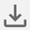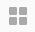From the Visualization Gallery, drag and drop the XY icon onto the plot.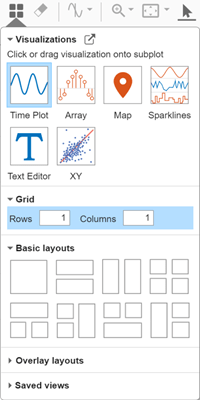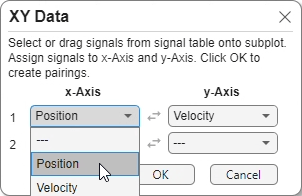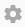To plot signals on the XY plot, select two signals and drag them onto the plot. For example, drag and drop the Heart-X and Heart-Y signals onto the plot. Specify which signal to use as x-data and which to use as y-data in the menu that appears in the lower-right of the plot.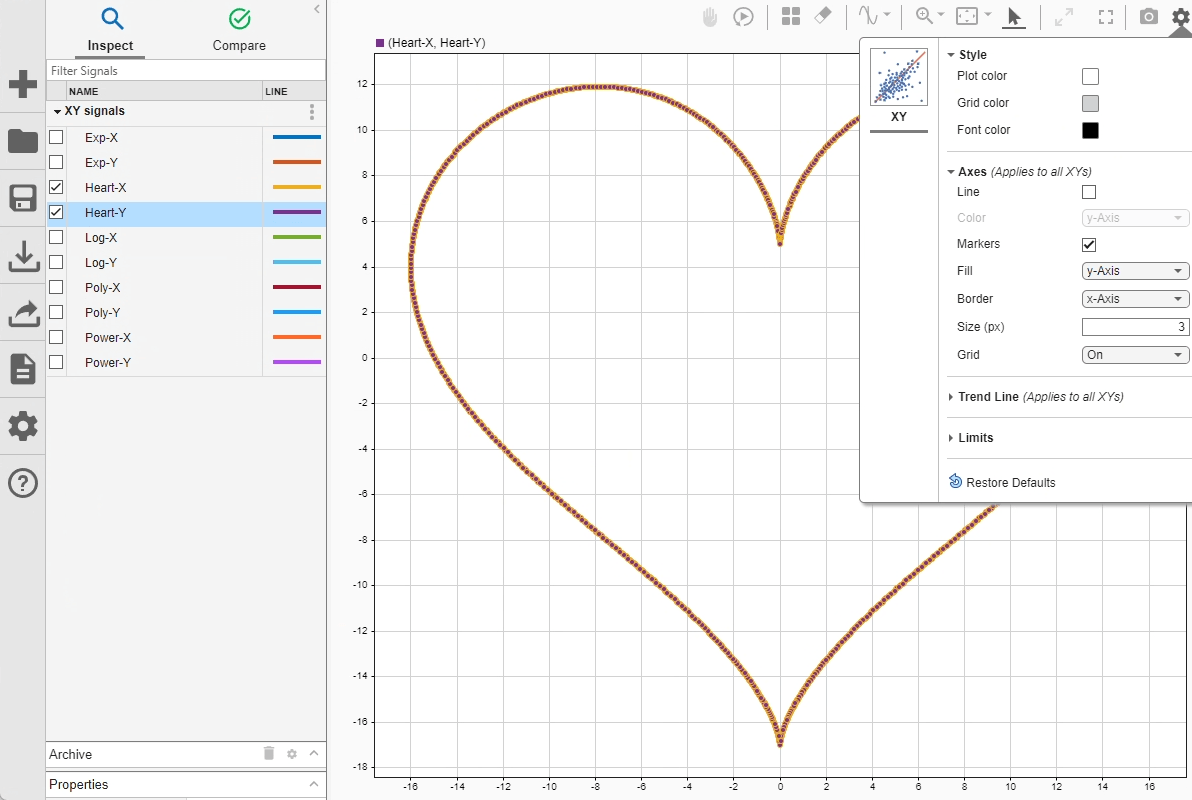You can customize the appearance of the XY plot using the Visualization Settings. The line and marker colors can match the color for the signal that provides the x data or the color for the signal that provides the y data. By default, the line and markers use the y-axis signal color. Specified settings apply for all XY plots in the layout.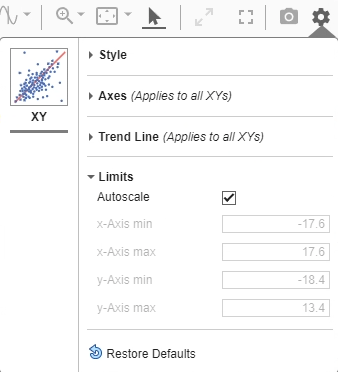Analyze XY Data Using a Trend Line

You can add a trend line to an XY plot to analyze the relationship between the x- and y-data. The trend line is most useful when the relationship can be expressed as a function. The Heart-X and Heart-Y data plotted in the prior section is not well-suited for trend line analysis because the y-data is not well correlated with the x-data.

The example data includes x- and y-data well-suited for each available type of trend line. You can try plotting each pair of signals to see how each trend line helps you analyze the data. For example, plot the Poly-X and Poly-Y signals on the XY visualization:

1. Select both signals.

2. Drag and drop the signals onto the plot.

3. Select Poly-X from the first list for series 1 and Poly-Y from the second list for series 1.

4. Click OK.

To add a trend line, open the Visualization Settings and select Show trend line. The default trend line type is Linear. From the Type list, select Polynomial.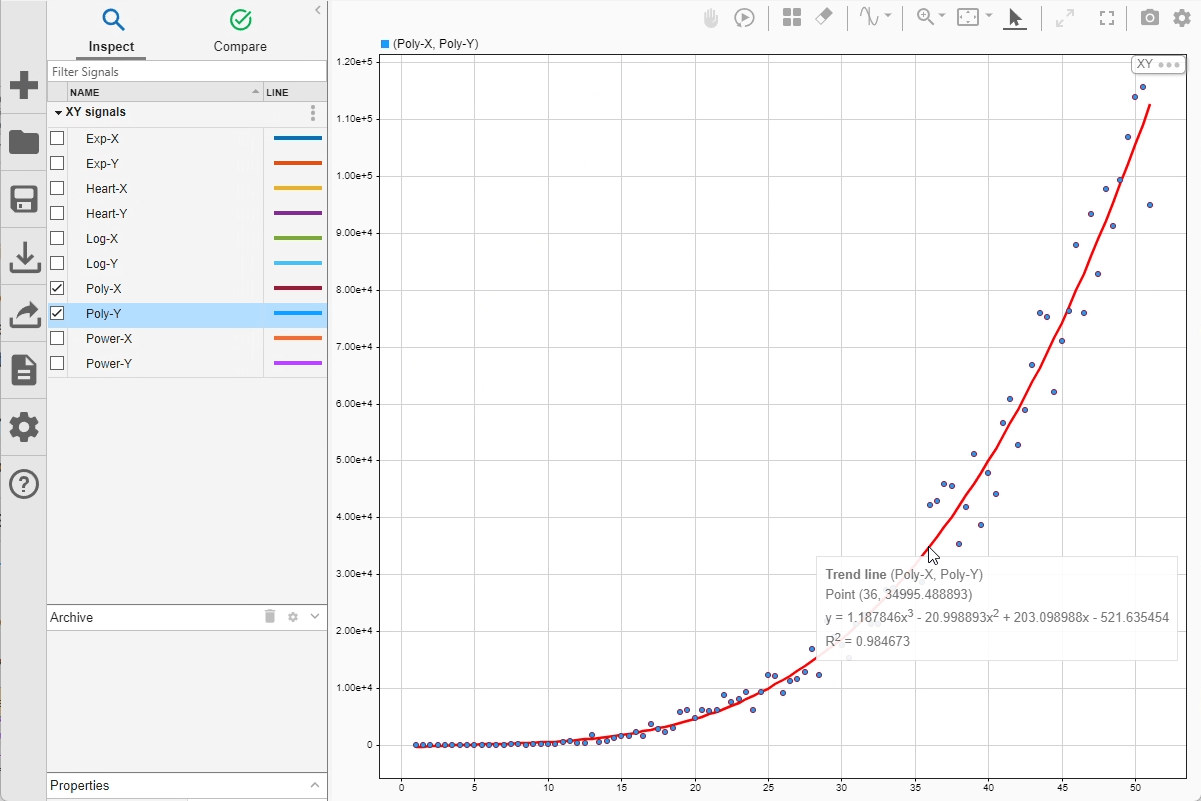By default, the Simulation Data Inspector computes the trend line in the form of a third-order polynomial. You can use the text box next to the selected trend line type to specify the order of the polynomial, between 2 and 6. Modify the order of the trend line polynomial to see how it affects the fit of the trend line. For example, when you change to use a second-order polynomial, the trend line does not fit the start of the data as well.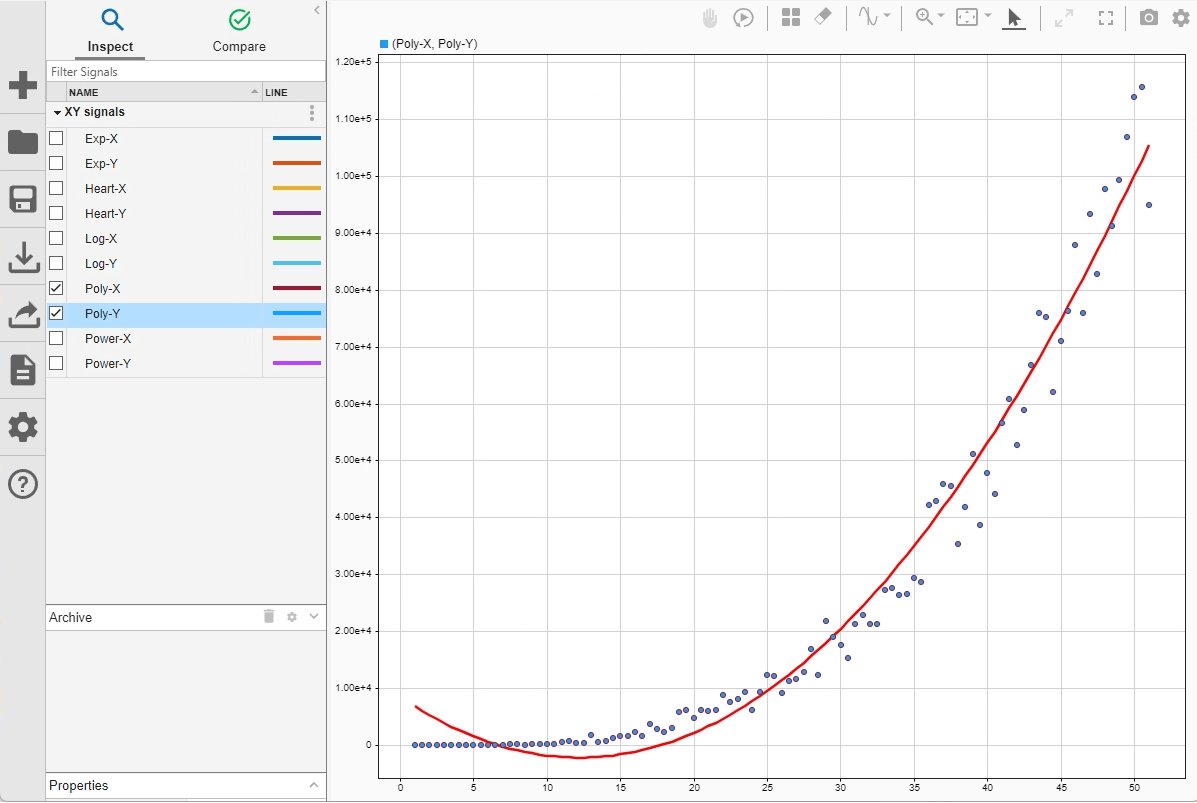Try out other trend line options to see how they fit the Poly-X and Poly-Y data. You can add these types of trend line to the XY plot:

• Linear: The trend line equation is of the form $\mathit{y}=\mathrm{mx}+\mathit{b}$.

• Logarithmic: The trend line equation is of the form $\mathit{y}=\mathit{a}\text{\hspace{0.17em}}\mathrm{ln}\left(\mathit{x}\right)+\mathit{b}$. The x-data must not contain 0 or negative values.

• Polynomial: The trend line equation is of the form $\mathit{y}={\mathit{b}}_{6}{\mathit{x}}^{6}...+{\mathit{b}}_{2}{\mathit{x}}^{2}+{\mathit{b}}_{1}\mathit{x}+\mathit{a}$, where the number of terms is determined by the specified order.

• Exponential: The trend line equation is of the form $\mathit{y}=\mathit{a}{\mathit{e}}^{\mathit{bx}}$. The y-data must not contain 0 or negative values.

• Power: The trend line equation is of the form $\mathit{y}=\mathit{a}{\mathit{x}}^{\mathit{b}}$. The x- and y-data must not contain 0 or negative values.

Try plotting other pairs of signals in the XY plot and explore how each trend line option fits each data set.